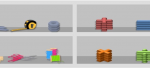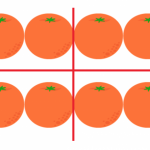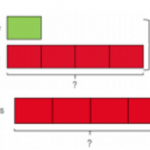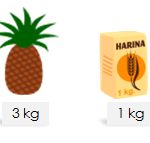Smartick is an online platform for children to master math in only 15 minutes a day

# I want to learn about: Word Problems

Learn mathematics word problems with Smartick. Activities, exercises, tutorials, and word problems for elementary aged children. Here you will find posts about word problems to make learning mathematics easier and more fun.

Sep06

## Solve Fraction Problems with Halves, Thirds and QuartersVery often we find, both in mathematical problems and in real life, fraction phrases like: a half, a third or a quarter. They are the most famous fractions! I’m sure you’ve seen them before. If you want to see what they are and how to calculate them, you can check out this link: Calculating halves, thirds […]

Jul19

## How to Solve a Multiplication ProblemDo you want to know how to solve a multiplication problem? Have you already learned how to multiply? Did you know that solving problems is the best way to learn multiplication? That’s why we are going to look at the different steps that we need to follow in order to solve a multiplication problem. Let’s […]

Jun24

## Mathematical Problems and Their Semantic CategoriesHow strange is the wording of these problems! The justification of these convoluted statements that seem like a bad idea by the author. It has always been so, that when we teach mathematics there are two large areas: that of “exercises” and that of “problems“. Exercises could be defined as activities to practice how tools […]

May05

## What do people mean by “a Quarter”?Have you ever been told or have you ever read, “It was a quarter of the class,” or “It took me a quarter of what I thought,” or “I have an attendance rate of 25%.”  What do we mean by “quarter?” Today we will discuss a quarter: What it is and what we use it […]

Mar20

## Practice Solving Multiplication Problems

This post will teach you how to analyze, process and solve problems that can be solved by using multiplication. At Smartick, we believe that a student understands and knows math when they can solve problems correctly. This is why we are going to analyze five different multiplication problems. Multiplication Problem #1 At Ricardo’s farm, there […]

Feb06

## Learn How to Solve a Time Word ProblemIn today’s post, we are going to work with time word problems. We’ll take a look at some examples and solve them together. Let’s begin! Time Word Problem 1: The Sailboat Race In a sailboat race, the winning boat completed two distances in the following times: 2 min 22 seconds and 3 min 45 seconds.  How […]

Jan27

## Learn How to Do Division Word Problems with DecimalsToday we are going to review division word problems with decimals. Does it seem difficult? Well, it’s not! Solving division word problems with decimals is the same as solving them with whole numbers. I’m going to show you! You can review what a decimal number is here. Every time we solve a word problem, the first […]

Dec01

## Singapore Method: Using the Singapore Bar Models to Solve ProblemsIn previous posts, we’ve talked about how to use the Singapore Method with different math operations. In today’s post, we’re going to look at what kinds of advantages this method boasts for solving problems. We know that problem solving is one of the most important parts of math. So, we need to provide students with tools […]

Nov07

## Mass Measurement ProblemsIn the U.S. the most common units for measuring mass are the ounce (oz) and the pound (lb). Technically, the pound is a measure of force, not mass, and the ounce can be a unit of volume or weight, which makes things even more confusing! But I won’t nitpick here! What is more important is […]